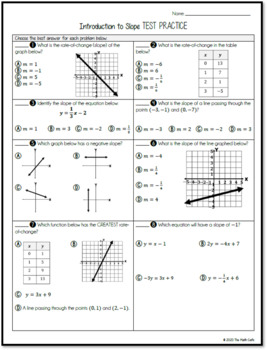# Introduction to Slope (NOTES, PRACTICE, QUICK DRILL, TEST PRACTICE)Subject
Resource Type
File Type

Zip

(1 MB|19+)
Product Rating
Standards
Also included in:
1. This resource was developed to partially meet the requirements of the 8th Grade Functions Standards below, with primary focus on SLOPE/RATE OF CHANGE:CCSS.MATH.CONTENT.8.F.A.2Compare properties of two functions each represented in a different way (algebraically, graphically, numerically in tables, o
\$7.50
\$5.00
Save \$2.50
• Product Description
• StandardsNEW

This resource was developed to partially meet the requirements of the 8th Grade Functions Standards below, with primary focus on SLOPE/RATE OF CHANGE:

CCSS.MATH.CONTENT.8.F.A.2
Compare properties of two functions each represented in a different way (algebraically, graphically, numerically in tables, or by verbal descriptions). For example, given a linear function represented by a table of values and a linear function represented by an algebraic expression, determine which function has the greater rate of change.

CCSS.MATH.CONTENT.8.F.B.4
Construct a function to model a linear relationship between two quantities. Determine the rate of change and initial value of the function from a description of a relationship or from two (x, y) values, including reading these from a table or from a graph. Interpret the rate of change and initial value of a linear function in terms of the situation it models, and in terms of its graph or a table of values.

It contains the following resources:

1) Introduction to Slope NOTES & PRACTICE PROBLEMS

· Types of Slopes QUICK DRILL* [Display in Separate PPT File]

· Graphs

· Tables

· Coordinates

· Equations**

· Comparing Slopes in Different Contexts

2) Multiple Choice TEST PRACTICE

3_ Answer Keys to All Parts

*A version of the Quick Drill for Google Forms included

**Equations: There is an OPTIONAL challenge included which teaches students how to convert an equation in standard form to slope-intercept. However, this skill is NOT found anywhere in the 8th grade standards and is introduced in 9th grade.

LICENSING TERMS: This purchase includes a license for one teacher only for personal use in their classroom. Licenses are non-transferable, meaning they can not be passed from one teacher to another. No part of this resource is to be shared with colleagues or used by an entire grade level, school, or district without purchasing the proper number of licenses.

COPYRIGHT TERMS: This resource may not be uploaded to the internet in any form, including classroom/personal websites or network drives, unless the site is password protected and can only be accessed by students.

Construct a function to model a linear relationship between two quantities. Determine the rate of change and initial value of the function from a description of a relationship or from two (𝘹, 𝘺) values, including reading these from a table or from a graph. Interpret the rate of change and initial value of a linear function in terms of the situation it models, and in terms of its graph or a table of values.
Compare properties of two functions each represented in a different way (algebraically, graphically, numerically in tables, or by verbal descriptions). For example, given a linear function represented by a table of values and a linear function represented by an algebraic expression, determine which function has the greater rate of change.
Total Pages
19+
Included
Teaching Duration
2 days
Report this Resource to TpT
Reported resources will be reviewed by our team. Report this resource to let us know if this resource violates TpT’s content guidelines.